## Making Math Easy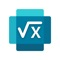# Microsoft Math Solver - HW app

by Microsoft Corporation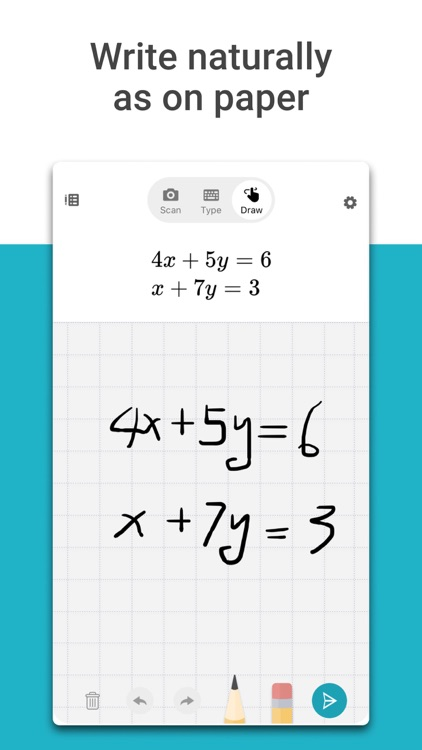Learn math with FREE step-by-step instructions. Get homework help for simple Arithmetic to advanced Algebra & Calculus. Scan math photo, use handwriting or calculator. Microsoft Math instantly recognizes the problem and helps you to solve it with detailed step-by-step explanation, interactive graphs, similar problems from the web and online video lectures. Quickly look up related math concepts. Gain confidence in mastering the techniques with Microsoft Mathematics. It is absolutely FREE and NO ads!### App Details

Version
1.0.23
Rating
(27270)
Size
53Mb
Genre
Education Utilities
Last updated
March 17, 2021
Release date
November 21, 2019

### App Screenshots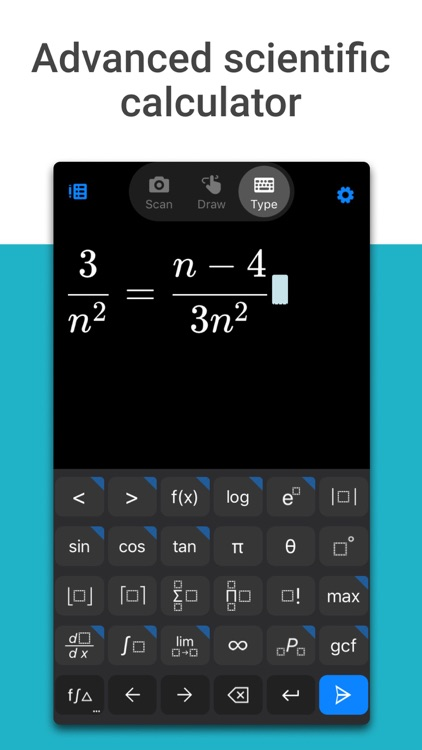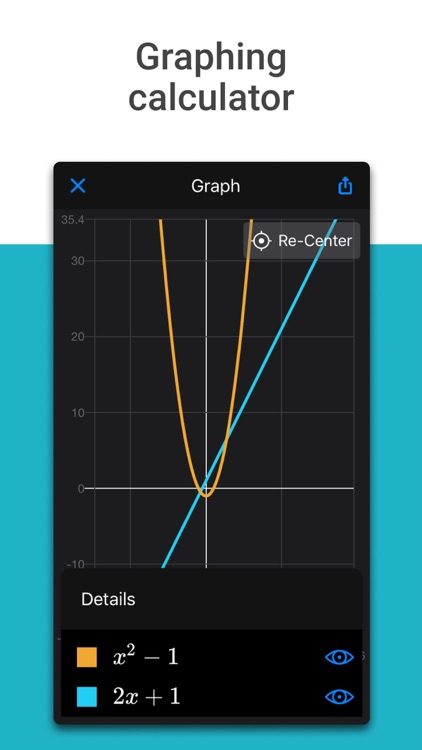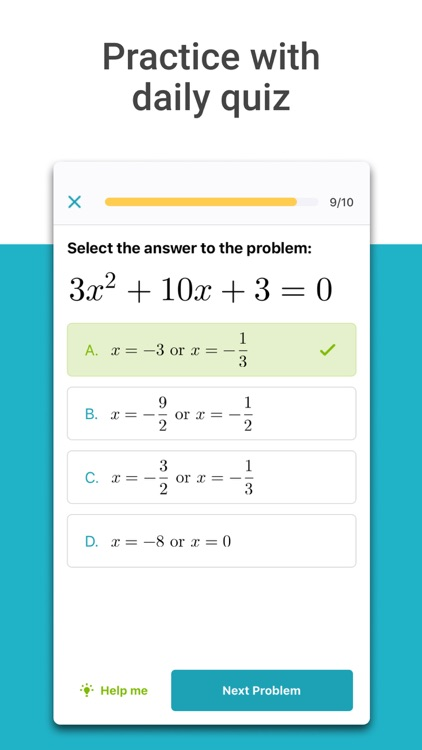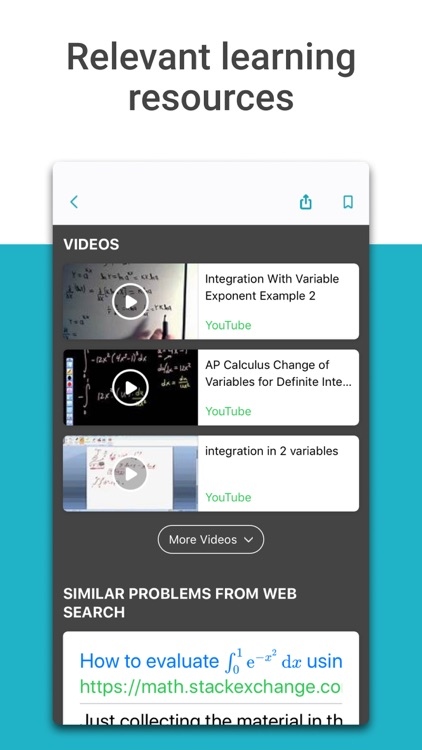### App Store Description

Learn math with FREE step-by-step instructions. Get homework help for simple Arithmetic to advanced Algebra & Calculus. Scan math photo, use handwriting or calculator. Microsoft Math instantly recognizes the problem and helps you to solve it with detailed step-by-step explanation, interactive graphs, similar problems from the web and online video lectures. Quickly look up related math concepts. Gain confidence in mastering the techniques with Microsoft Mathematics. It is absolutely FREE and NO ads!

MAIN FEATURES
● Write a math equation on screen as you naturally do on paper
● Scan printed or handwritten math photo
● Type and edit using advanced scientific math calculator
● Get interactive Step-by-Step explanation & Graphing calculator
● Import images with math equations from gallery
● Scan and Solve Math Worksheets with multiple problems
● Search the web for similar problems and video lectures
● Try math word problems
● Scan and plot x-y data tables for linear/non-linear functions
● Learn math in your language - supports Chinese, French, German, Hindi, Italian, Japanese, Portuguese, Russian, Spanish, and many more

SUPPORTED PROBLEMS
● Elementary: arithmetic, real, complex numbers, LCM, GCD, factors, roman numerals
● Pre-Algebra: radicals and exponents, fractions, matrices, determinants
● Algebra: quadratic equations, system of equations, inequalities, rational expressions, linear, quadratic and exponential graphs
● Word problems on math concepts, number theory, probability, volume, surface area
● Basic Calculus: Summations, Limits, derivatives, integrals
● Statistics: Mean, Median, Mode, Standard Deviation, permutations, combinations

Microsoft Math solver combines the power of multiple solvers such as math problem solver, equation solver, math answer scanner, percentage calculator, scientific calculator, word problem solver, math photo solver and math handwriting solver. This is a powerful math learner app for solving math homework problems. Find out more about Microsoft Math Solver app on our website: https://math.microsoft.com